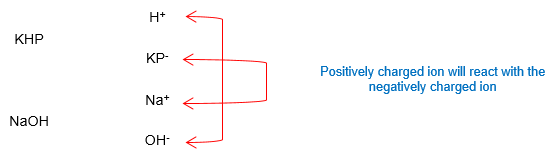# Problem: Potassium hydrogen phthalate (KHP) is often used as a primary standard in acid-base titrations. If 19.15 mL of NaOH is required to neutralize 0.442 g of KHP, what is the concentration of NaOH?

79% (90 ratings)
###### FREE Expert Solution

During titration, the acid and base reacts. In the given, NaOH is the base while KHP is the acid. Since KHP is an acid, it will donate a proton (H+) (based on the Bronsted-Lowry definition).

KHP and NaOH will break up into their ions in the solution and will react as shown:Balanced Reaction:  KHP + NaOH  NaKP + H2O

Given:

V NaOH = 19.15 mL

Mass KHP = 0.442 g

[NaOH] = ?

Calculate for the concentration of NaOH using the following steps:

79% (90 ratings)###### Problem Details

Potassium hydrogen phthalate (KHP) is often used as a primary standard in acid-base titrations. If 19.15 mL of NaOH is required to neutralize 0.442 g of KHP, what is the concentration of NaOH?

What scientific concept do you need to know in order to solve this problem?

Our tutors have indicated that to solve this problem you will need to apply the Solution Stoichiometry concept. You can view video lessons to learn Solution Stoichiometry. Or if you need more Solution Stoichiometry practice, you can also practice Solution Stoichiometry practice problems.

What is the difficulty of this problem?

Our tutors rated the difficulty ofPotassium hydrogen phthalate (KHP) is often used as a primar...as medium difficulty.

How long does this problem take to solve?

Our expert Chemistry tutor, Sabrina took 5 minutes and 20 seconds to solve this problem. You can follow their steps in the video explanation above.

What professor is this problem relevant for?

Based on our data, we think this problem is relevant for Professor Santander's class at TAMU.